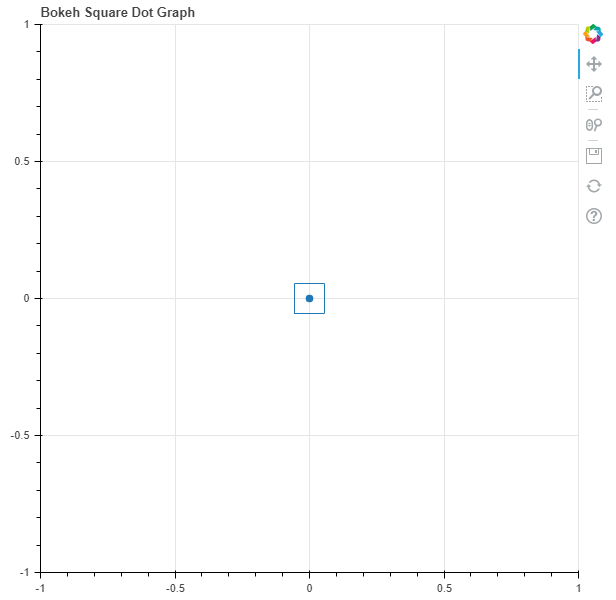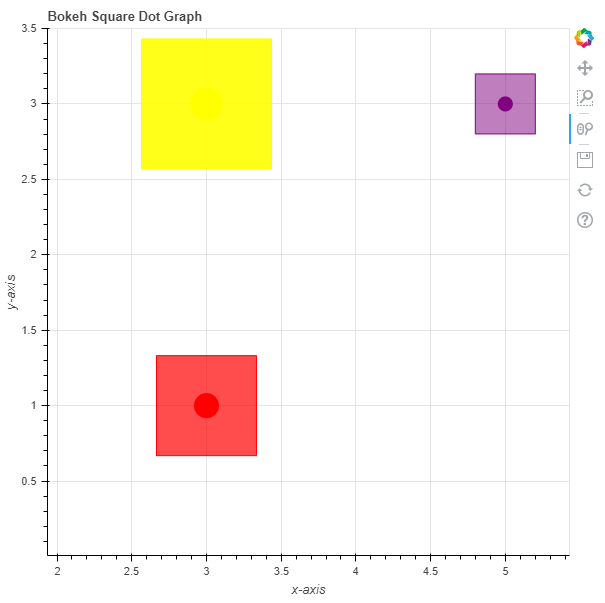Related Articles

# Python Bokeh – Plotting Squares with Dots on a Graph

• Last Updated : 10 Jul, 2020

Bokeh is a Python interactive data visualization. It renders its plots using HTML and JavaScript. It targets modern web browsers for presentation providing elegant, concise construction of novel graphics with high-performance interactivity.

Bokeh can be used to plot squares with dots on a graph. Plotting squares with dots on a graph can be done using the `square_dot()` method of the `plotting` module.

## plotting.figure.square_dot()

Syntax : square_dot(parameters)

Parameters :

• x : x-coordinates of the center of the square
• y : y-coordinates of the center of the square

Returns : an object of class `GlyphRenderer`

Example 1 : In this example we will be using the default values for plotting the graph.

 `# importing the modules``from` `bokeh.plotting ``import` `figure, output_file, show``       ` `# file to save the model``output_file(``"gfg.html"``)``       ` `# instantiating the figure object``graph ``=` `figure(title ``=` `"Bokeh Square Dot Graph"``)``     ` `# the points to be plotted``x ``=` `0``y ``=` `0``    ` `# plotting the graph``graph.square_dot(x, y, size ``=` `30``, fill_color ``=` `None``)``     ` `# displaying the model``show(graph)`

Output :Example 2 : In this example we will be plotting the multiple squares with various other parameters

 `# importing the modules ``from` `bokeh.plotting ``import` `figure, output_file, show `` ` `# file to save the model ``output_file(``"gfg.html"``) ``      ` `# instantiating the figure object ``graph ``=` `figure(title ``=` `"Bokeh Square Dot Graph"``) `` ` `# name of the x-axis ``graph.xaxis.axis_label ``=` `"x-axis"``      ` `# name of the y-axis ``graph.yaxis.axis_label ``=` `"y-axis"`` ` `# points to be plotted``x ``=` `[``3``, ``3``, ``5``]``y ``=` `[``3``, ``1``, ``3``]``size ``=` `[``130``, ``100``, ``60``]`` ` `# color value of the square``color ``=` `[``"yellow"``, ``"red"``, ``"purple"``]`` ` `# fill alpha value of the square``fill_alpha ``=` `[``0.9``, ``0.7``, ``0.5``]`` ` `# plotting the graph ``graph.square_dot(x, y,``                 ``size ``=` `size,``                 ``color ``=` `color,``                 ``fill_alpha ``=` `fill_alpha) ``      ` `# displaying the model ``show(graph)`

Output :Attention geek! Strengthen your foundations with the Python Programming Foundation Course and learn the basics.

To begin with, your interview preparations Enhance your Data Structures concepts with the Python DS Course. And to begin with your Machine Learning Journey, join the Machine Learning – Basic Level Course

My Personal Notes arrow_drop_up